# Perimeter + unit conversion - math problems

#### Number of problems found: 57

• The homeowner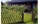The homeowner plans to build a fence along the sides and back of the property. How many feet of fencing are required to build the fence? (1 metre = 3.2808399 feet)Lots: 14w x 36.6d (m)
• Minute handWhat is the distance the minute hand of the clock travels in 12 minutes, if the diameter of the clock is 30 cm and the hand extends to a distance of 2 cm from the edge of the clock?
• Perimeter of the park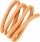Find the perimeter of the park in meters in a diamond shape, if the length of its side is 2 km.
• A bicycle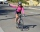A bicycle wheel has a diameter of 63 cm. Calculate how many times the wheel turns round in travelling 19.8 km .
• The parallelogram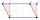The parallelogram has one side 2 dm long, which is one-sixth of its circumference. How many cm does the other side of the parallelogram measure?
• A bug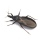A bug was sitting on the tip of a wind turbine blade that was 24 inches long when it started to rotate. The bug held on for five rotations before flying away. How far did the bug travel before it flew off? Exact answer.
• Orange and tangerine treesBrad's father asked an engineer to survey the field behind their house. He wanted to plant some orange and tangerine trees there. According to the survey, the field is thirty-two feet long and six yards wide. What is the area of the field in sq feet?
• The central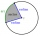The central angle of a sector is 30° and the radius is 15 m. Determine its perimeter.
• GearsThe front gear on the bike has 32 teeth and the rear, on the wheel, has 12 teeth. How many times does the bike's rear-wheel turn if you turn the right pedal 30 times? What distance will you go if the circumference of the bicycle wheel is 250 cm?
• How many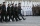How many 75 cm long steps will a football player take during training, when he runs on a 50m square track and goes around the course 3 times? We need writing, sketch, calculation. .. .
• Central angleWhat is the length of the arc of a circle with a diameter of 46 cm, which belongs to a central angle of 30°?
• Squares ratioThe first square has a side length of a = 6 cm. The second square has a circumference of 6 dm. Calculate the proportions of the perimeters and the proportions of the contents of these squares? (Write the ratio in the basic form). (Perimeter = 4 * a, conte
• TableclothsThe restaurant has sixty-two square tablecloths with a side length of 150 cm and 36 rectangular tablecloths with dimensions of 140 cm and 160 cm. A) How many meters of hemming ribbon will be needed if we add 50 cm to each tablecloth? B) The ribbon sale in
• Circular railwayThe railway connects in a circular arc the points A, B, and C, whose distances are | AB | = 30 km, AC = 95 km, BC | = 70 km. How long will the track from A to C?
• Velocipedes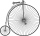In the 19th century, bicycles did not have a chain drive, and the wheel axis connected the pedals directly. This wheel diameter gradually increased until the so-called high bikes (velocipedes) with a front-wheel diameter of up to 1.5 meters, while the rea
• DecagonCalculate the area and circumference of the regular decagon when its radius of a circle circumscribing is R = 1m
• The small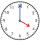The small hand of the wall clock is 15 cm long. What a long journey the end of the hour hand in 15 minutes will describe. (b - in 2 hours)?
• RectanglesHow many different rectangles with sides integers (in mm) have a circumference exactly 1000 cm? (a rectangle with sides of 50cm and 450cm is considered to be the same as a rectangle with sides of 450cm and 50cm)
• Christmas napkinsThe girls embroidered Christmas napkins. Each napkin had the shape of a triangle with sides of 5 dm, 60 cm, and 800 mm. How many cms did the girls sew if they made 15 napkins?
• The sidesThe sides of a rectangle are in a ratio of 2:3, and its perimeter is 1 1/4 inches. What are the lengths of its side? Draw it.

Do you have an exciting math question or word problem that you can't solve? Ask a question or post a math problem, and we can try to solve it.

We will send a solution to your e-mail address. Solved examples are also published here. Please enter the e-mail correctly and check whether you don't have a full mailbox.

Perimeter - math problems. Unit conversion - math problems.